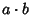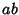## Multiplication

In simple algebra, multiplication is the process of calculating the result when a numberis takentimes. The result of a multiplication is called the Product ofand. It is denoted,,, or simply. The symbolis known as the Multiplication Sign. Normal multiplication is Associative, Commutative, and Distributive.

More generally, multiplication can also be defined for other mathematical objects such as Groups, Matrices, Sets, and Tensors.

Karatsuba and Ofman (1962) discovered that multiplication of twodigit numbers can be done with a Bit Complexity of less thanusing an algorithm now known as Karatsuba Multiplication.

See also Addition, Bit Complexity, Complex Multiplication, Division, Karatsuba Multiplication, Matrix Multiplication, Product, Russian Multiplication, Subtraction, Times

References

Karatsuba, A. and Ofman, Yu. Multiplication of Many-Digital Numbers by Automatic Computers.'' Doklady Akad. Nauk SSSR 145, 293-294, 1962. Translation in Physics-Doklady 7, 595-596, 1963.